/

### Extreme Events – Specimen Question A.2.3(a) – Answer/Hints

Q. Recalculate the mean, standard deviation, skew and kurtosis weighting the data as above. Are they still suggestive of fat-tailed behaviour?

The proposed exponential weighting approach gives the following weights to the observations:

 Period Weight A (logged return) 1 0.2679434 -0.2890163 2 0.2871746 0.0009995 3 0.3077861 0.2167230 4 0.3298770 0.0760347 5 0.3535534 -0.0140989 6 0.3789291 0.0049875 7 0.4061262 -0.0060181 8 0.4352753 0.0099503 9 0.4665165 0.0704585 10 0.5 -0.1278334 11 0.5358867 0.0069756 12 0.5743492 0.0079682 13 0.6155722 -0.0387408 14 0.6597540 0.0276152 15 0.7071068 -0.0439519 16 0.7578583 -0.0120726 17 0.8122524 -0.0725707 18 0.8705506 0.0629748 19 0.9330330 0.0353671 20 1 0.0178399

The ordinary mean of a set of numbers is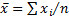, where the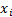are the observations and there areof them. The weighted mean, if each observation is given a weight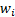is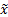, say, where: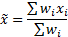We see immediately that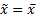if the observations are given equal weight, i.e. if all theare equal.

It is relatively simple to calculate this statistic from the above data using Microsoft Excel (or using the Nematrian web function MnWeightedMean), giving an answer of -0.0007543

However, identifying exactly how to calculate the corresponding weighted standard deviation, skew and kurtosis is less simple, particularly if our focus is on small samples.

For ‘population’ statistics, i.e. those applicable if we have large observation sets, the calculations involved appear to be relatively unambiguous, see WeightedMomentsAndCumulants

However, for ‘sample’ statistics, there appear to be differences in opinion in how, precisely, to adjust ‘population’ statistics to allow for the different degrees of freedom that are present. Given this apparent disagreement between commentators, the Nematrian website provides web functions for calculating the weighted population mean, standard deviation, skew and excess (excess) kurtosis, but only corresponding weighted sample mean, standard deviation and skew (i.e. not yet weighted sample excess kurtosis). Rimoldini (2013) proposes some formulae for these statistics, which appear to match the Nematrian formulae.

Formulae used by the Nematrian website can be accessed using the following web functions: MnWeightedPopulationStdev, MnWeightedPopulationSkew, MnWeightedPopulationKurt, MnWeightedStdev and MnWeightedSkew and give the following results for the above data:

 Statistic Unweighted (population) Unweighted (sample) Weighted (population) Weighted (sample) Mean -0.0033204 -0.0033204 -0.0007543 -0.0007543 Standard deviation 0.0930001 0.0954161 0.0741301 0.0763675 Skew -0.791166 -0.9253403 -0.8903571 -0.9761716 (Excess) kurtosis 2.6500366 4.6238918 5.0848899 N/A

These statistics are still strongly suggestive of fat-tailed behaviour.